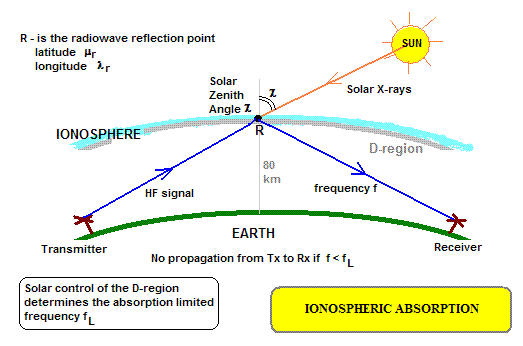### Solar X-ray Induced Ionospheric Absorption

INTRODUCTION

This model allows the computation of the maximum absorption frequency due to solar X-ray control of the ionospheric D-layer. This can be used to infer the extent of a shortwave fadeout in high frequency (HF) communications. It can also be used to infer outages of Over the Horizon radar (OTHR) systems. In both cases the model can be used for frequency management.

During daytime hours the ionosphere contains a structure called the D-region. This is a region of solar-produced ionisation around 80 km altitude. Unlike the E and F layers above it, the D-region does not reflect radio signals, but it does absorb them. The frequency below which absorption becomes significant is called the absorption limited frequency fL (also sometimes the ALF).

During quiet solar times, most of the D-region ionisation is produced by Lyman-alpha (Lα radiation with a wavelenth of 121.6 nm. However, when a solar flare occurs the ionisation increase due to the action of soft solat x-rays in the wavelength range from 0.1 to 0.8 nm. These x-rays are monitored by sensors on the US GOES satellites and are available in real-time from the SWPC web site.Solar soft x-ray flares in the 0.1 to 0.8 nm wavelength range have energy flux densities that are assigned letters followed by a number. The letters indicate the class of the flare (C=low flux, M=moderate flux, X=high flux). The number then indicates the flux density within this specified decadal range. At times of low solar activity, the letters B and A are used for the decadal ranges below C, and very energetic flares are sometimes classed as Y flares where Y = 10*X. Thus an X28 flare is also known as a Y2.8 flare. Note that the GOES detectors saturate at about X17 and any flare classed >X17 has been assigned by extrapolation of the actual flux curve.

ABSORPTION MODEL

The absorption of radio waves occurs where they pentrate the D-region. For sub-ionospheric waves this occurs in two places, one where they are ascending and the other where they are descending. We simplify reality in this model by assuming a single absorptive location at the ionospheric reflection point (R). The situation is indicated in the diagram below:If the ray requires more than one hop to reach the receiver from the transmitter, the reflection point that has the most absorption will be the one controlling the propagation.

The absorption by the D-region is dependent on the solar zenith angle ( χ ) at point R, and the absorption limited frequency may be expressed empirically (from work carried out at the US Naval Ocean Systems Center) by the model formula:

fL = 174 ( X + 1.8 x 10-6 )1/4 cos3/4( χ )
where X is the solar X-ray flux in Watts per square metre. The second termed in the parenthese is due to Lyman-α radiation. This can be seem to be equivalent to C1.8 x-ray emission. It is this term that generally controls the absorption when no solar flare is occurring.

APRROXIMATIONS

To compute the solar zenith angle at point R we use the formula:

χ = cos-1 [ sin ( μR ) sin ( δ ) + cos ( μR ) cos ( δ ) cos ( λS - λR ) ]
where
μR is the reflection point (R) latitude
λR is the relection point (R) longitude
δ is the solar declination
λS is the solar longitude
For the purposes of this model the last two quantities can be approximated by the simple expressions:
λSo = 15 ( 12 - UT )
δo = 23.5 sin ( YD - 82 )o
where
UT is the universal time in hours ( 0 - 23.9 )
YD is the day of the year ( 1 to 365 )
MODEL I/O

INPUTS

The model inputs are the location of the ionospheric reflection point (latitude and longitude), the date (day of year) and the time (Universal Time UT).

OUTPUTS

The model output is the absorption limited frequency fL for the specified reflection point and date and time.

IMPLEMENTATION

A Quick Basic implementation of this model may be found at SWF.BAS with an accompanying world map file at WS.MAP. An output map produced by this program is shown below:This plot shows the lowest useable frequencies for ionospheric reflection points across the globe during an X2 solar X-ray flare at a time of 00 UT hours on the 23rd of October. The colour bar on the right specifies the ALF frequencies appropriate for each colour. Note that only the daylight side of the Earth is affected.

The following graph is an empirical plot of the maximum frequency predicted by the model and in addition the average duration that a shortwave fadeout lasts for each class of X-ray flare. This is based on a model developed at the USAF Air Weather Service in 1988.Australian Space Academy